# Embedded Images Quiz I, Non Verbal Reasoning

## 25 Questions MCQ Test Non Verbal Reasoning | Embedded Images Quiz I, Non Verbal Reasoning

Description
Attempt Embedded Images Quiz I, Non Verbal Reasoning | 25 questions in 50 minutes | Mock test for LR preparation | Free important questions MCQ to study Non Verbal Reasoning for LR Exam | Download free PDF with solutions
QUESTION: 1

### Directions to Solve In each of the following questions, you are given a figure (X) followed by four alternative figures (1), (2), (3) and (4) such that figure (X) is embedded in one of them. Trace out the alternative figure which contains fig. (X) as its part. Question - Find out the alternative figure which contains figure (X) as its part.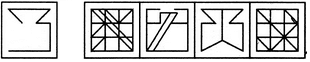(X)                (1)         (2)         (3)        (4)

Solution: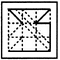QUESTION: 2

### Directions to Solve In each of the following questions, you are given a figure (X) followed by four alternative figures (1), (2), (3) and (4) such that figure (X) is embedded in one of them. Trace out the alternative figure which contains fig. (X) as its part. Question - Find out the alternative figure which contains figure (X) as its part.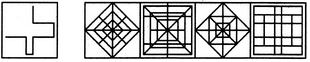(X)                (1)         (2)         (3)        (4)

Solution: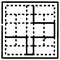QUESTION: 3

### Directions to Solve In each of the following questions, you are given a figure (X) followed by four alternative figures (1), (2), (3) and (4) such that figure (X) is embedded in one of them. Trace out the alternative figure which contains fig. (X) as its part. Question - Find out the alternative figure which contains figure (X) as its part.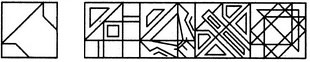(X)                (1)         (2)         (3)        (4)

Solution: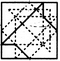QUESTION: 4

In each of the following questions, you are given a figure (X) followed by four alternative figures (1), (2), (3) and (4) such that figure (X) is embedded in one of them. Trace out the alternative figure which contains fig. (X) as its part.

Question -

Find out the alternative figure which contains figure (X) as its part.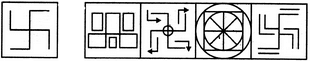(X)                (1)         (2)         (3)        (4)
Solution: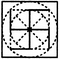QUESTION: 5

In each of the following questions, you are given a figure (X) followed by four alternative figures (1), (2), (3) and (4) such that figure (X) is embedded in one of them. Trace out the alternative figure which contains fig. (X) as its part.

Question -

Find out the alternative figure which contains figure (X) as its part.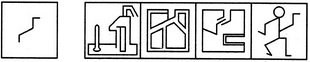(X)                (1)         (2)         (3)        (4)
Solution: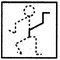QUESTION: 6

In each of the following questions, you are given a figure (X) followed by four alternative figures (1), (2), (3) and (4) such that figure (X) is embedded in one of them. Trace out the alternative figure which contains fig. (X) as its part.

Question -

Find out the alternative figure which contains figure (X) as its part.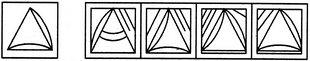(X)                (1)         (2)         (3)        (4)
Solution: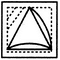QUESTION: 7

In each of the following questions, you are given a figure (X) followed by four alternative figures (1), (2), (3) and (4) such that figure (X) is embedded in one of them. Trace out the alternative figure which contains fig. (X) as its part.

Question -

Find out the alternative figure which contains figure (X) as its part.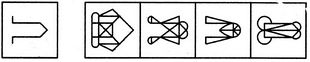(X)                (1)         (2)         (3)        (4)
Solution: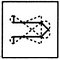QUESTION: 8

In each of the following questions, you are given a figure (X) followed by four alternative figures (1), (2), (3) and (4) such that figure (X) is embedded in one of them. Trace out the alternative figure which contains fig. (X) as its part.

Question -

Find out the alternative figure which contains figure (X) as its part.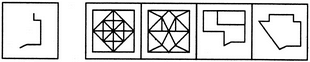(X)                (1)         (2)         (3)        (4)
Solution: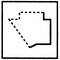QUESTION: 9

In each of the following questions, you are given a figure (X) followed by four alternative figures (1), (2), (3) and (4) such that figure (X) is embedded in one of them. Trace out the alternative figure which contains fig. (X) as its part.

Question -

Find out the alternative figure which contains figure (X) as its part.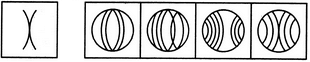(X)                (1)         (2)         (3)        (4)
Solution: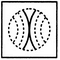QUESTION: 10

In each of the following questions, you are given a figure (X) followed by four alternative figures (1), (2), (3) and (4) such that figure (X) is embedded in one of them. Trace out the alternative figure which contains fig. (X) as its part.

Question -

Find out the alternative figure which contains figure (X) as its part.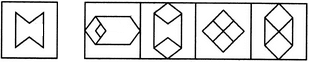(X)                (1)         (2)         (3)        (4)
Solution: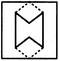QUESTION: 11

In each of the following questions, you are given a figure (X) followed by four alternative figures (1), (2), (3) and (4) such that figure (X) is embedded in one of them. Trace out the alternative figure which contains fig. (X) as its part.

Question -

Find out the alternative figure which contains figure (X) as its part.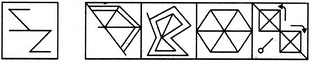(X)                (1)         (2)         (3)        (4)
Solution: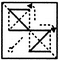QUESTION: 12

In each of the following questions, you are given a figure (X) followed by four alternative figures (1), (2), (3) and (4) such that figure (X) is embedded in one of them. Trace out the alternative figure which contains fig. (X) as its part.

Question -

Find out the alternative figure which contains figure (X) as its part.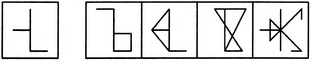(X)                (1)         (2)         (3)        (4)
Solution: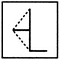QUESTION: 13

In each of the following questions, you are given a figure (X) followed by four alternative figures (1), (2), (3) and (4) such that figure (X) is embedded in one of them. Trace out the alternative figure which contains fig. (X) as its part.

Question -

Find out the alternative figure which contains figure (X) as its part.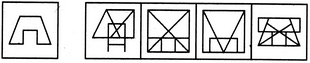(X)                (1)         (2)         (3)        (4)
Solution: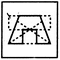QUESTION: 14

In each of the following questions, you are given a figure (X) followed by four alternative figures (1), (2), (3) and (4) such that figure (X) is embedded in one of them. Trace out the alternative figure which contains fig. (X) as its part.

Question -

Find out the alternative figure which contains figure (X) as its part.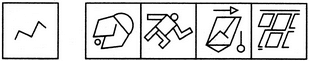(X)                (1)         (2)         (3)        (4)
Solution: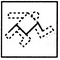QUESTION: 15

In each of the following questions, you are given a figure (X) followed by four alternative figures (1), (2), (3) and (4) such that figure (X) is embedded in one of them. Trace out the alternative figure which contains fig. (X) as its part.

Question -

Find out the alternative figure which contains figure (X) as its part.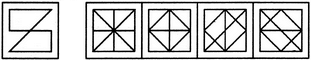(X)                (1)         (2)         (3)        (4)
Solution: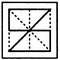QUESTION: 16

In each of the following questions, you are given a figure (X) followed by four alternative figures (1), (2), (3) and (4) such that figure (X) is embedded in one of them. Trace out the alternative figure which contains fig. (X) as its part.

Question -

Find out the alternative figure which contains figure (X) as its part.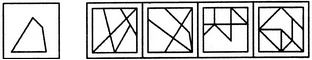(X)                (1)         (2)         (3)        (4)
Solution: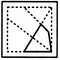QUESTION: 17

In each of the following questions, you are given a figure (X) followed by four alternative figures (1), (2), (3) and (4) such that figure (X) is embedded in one of them. Trace out the alternative figure which contains fig. (X) as its part.

Question -

Find out the alternative figure which contains figure (X) as its part.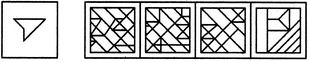(X)                (1)         (2)         (3)        (4)
Solution: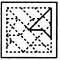QUESTION: 18

In each of the following questions, you are given a figure (X) followed by four alternative figures (1), (2), (3) and (4) such that figure (X) is embedded in one of them. Trace out the alternative figure which contains fig. (X) as its part.

Question -

Find out the alternative figure which contains figure (X) as its part.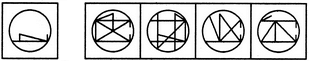(X)                (1)         (2)         (3)        (4)
Solution: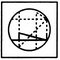QUESTION: 19

Directions to Solve

In each of the following questions, you are given a figure (X) followed by four alternative figures (1), (2), (3) and (4) such that figure (X) is embedded in one of them. Trace out the alternative figure which contains fig. (X) as its part.

Question -

Find out the alternative figure which contains figure (X) as its part.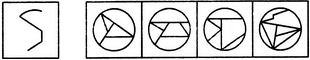(X)                (1)         (2)         (3)        (4)

Solution: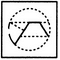QUESTION: 20

In each of the following questions, you are given a figure (X) followed by four alternative figures (1), (2), (3) and (4) such that figure (X) is embedded in one of them. Trace out the alternative figure which contains fig. (X) as its part.

Question -

Find out the alternative figure which contains figure (X) as its part.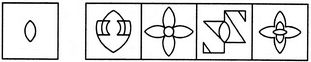(X)                (1)         (2)         (3)        (4)
Solution: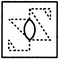QUESTION: 21

In each of the following questions, you are given a figure (X) followed by four alternative figures (1), (2), (3) and (4) such that figure (X) is embedded in one of them. Trace out the alternative figure which contains fig. (X) as its part.

Question -

Find out the alternative figure which contains figure (X) as its part.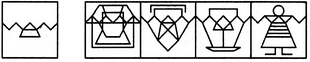(X)                (1)         (2)         (3)        (4)
Solution: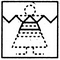QUESTION: 22

In each of the following questions, you are given a figure (X) followed by four alternative figures (1), (2), (3) and (4) such that figure (X) is embedded in one of them. Trace out the alternative figure which contains fig. (X) as its part.

Question -

Find out the alternative figure which contains figure (X) as its part.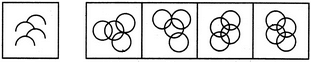(X)                (1)         (2)         (3)        (4)
Solution: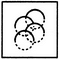QUESTION: 23

In each of the following questions, you are given a figure (X) followed by four alternative figures (1), (2), (3) and (4) such that figure (X) is embedded in one of them. Trace out the alternative figure which contains fig. (X) as its part.

Question -

Find out the alternative figure which contains figure (X) as its part.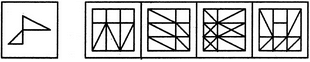(X)                (1)         (2)         (3)        (4)
Solution: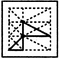QUESTION: 24

In each of the following questions, you are given a figure (X) followed by four alternative figures (1), (2), (3) and (4) such that figure (X) is embedded in one of them. Trace out the alternative figure which contains fig. (X) as its part.

Question -

Find out the alternative figure which contains figure (X) as its part.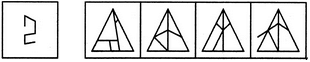(X)                (1)         (2)         (3)        (4)
Solution: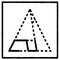QUESTION: 25

In each of the following questions, you are given a figure (X) followed by four alternative figures (1), (2), (3) and (4) such that figure (X) is embedded in one of them. Trace out the alternative figure which contains fig. (X) as its part.

Question -

Find out the alternative figure which contains figure (X) as its part.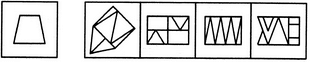(X)                (1)         (2)         (3)        (4)
Solution: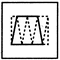Use Code STAYHOME200 and get INR 200 additional OFF Use Coupon Code